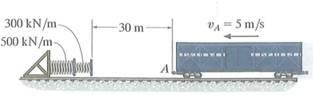Problem

# The train car has a mass of 10 Mg and is traveling at 5 m/s when it reaches A. If the roll...

The train car has a mass of 10 Mg and is traveling at 5 m/s when it reaches A. If the rolling resistance is 1/100 of the weight of the car, determine the compression of each spring when the car is momentarily brought to rest.#### Step-by-Step Solution

Solution 1

Calculate the rolling resistance.

$$F_{r}=\frac{1}{100} m g$$

Substitute $$10 \mathrm{Mg}$$ for $$m$$, and $$9.81 \mathrm{~m} / \mathrm{s}^{2}$$ for $$g$$.

\begin{aligned} F_{r} &=\frac{1}{100} \times 10 \times 10^{3} \times 9.81 \\ &=981 \mathrm{~N} \end{aligned}

Calculate the relationship between the distances of compression of each spring using conservation of energy as follows.

$$\frac{1}{2} m v^{2}=F_{r}\left(d+s_{1}+s_{2}\right)+\frac{1}{2} k_{1} s_{1}+\frac{1}{2} k_{2} s_{2}$$

Here, $$v$$ is the initial velocity of the train, $$d$$ is the initial distance of the train from the spring, $$s_{1}$$, is the compression in the spring 1 , and $$s_{2}$$ is the compression in the spring 2 .

Substitute $$10 \mathrm{Mg}$$ for $$m, 5 \mathrm{~m} / \mathrm{s}^{2}$$ for $$v, 30 \mathrm{~m}$$ for $$d, 300 \mathrm{kN} / \mathrm{m}$$ for $$k_{1}$$, and $$500 \mathrm{kN} / \mathrm{m}$$ for $$k_{2}$$.

\begin{aligned} &\frac{1}{2}\left(10 \times 10^{3}\right)(5)^{2}=981\left(30+s_{1}+s_{2}\right)+\frac{1}{2}\left(300 \times 10^{3}\right) s_{1}^{2}+\frac{1}{2}\left(500 \times 10^{3}\right) s_{2}^{2} \ldots \ldots (1)\\ &125000=981\left(30+s_{1}+s_{2}\right)+150000 s_{1}^{2}+250000 s_{2}^{2} \end{aligned}

Calculate the compression of each spring when the car is momentarily brought to rest by using principle of work and energy.

$$k_{1} s_{1}=k_{2} s_{2}$$

Substitute the known values in the above equation.

\begin{aligned} &\left(300 \times 10^{3}\right) s_{1}=\left(500 \times 10^{3}\right) s_{2} \ldots \ldots(2) \\ &s_{1}=1.666 s_{2} \end{aligned}

Substitute the above value in equation (1).

\begin{aligned} &125000=981\left(30+\left(1.666 s_{2}\right)+s_{2}\right)+150000\left(1.666 s_{2}\right)^{2}+250000 s_{2}^{2} \\ &125000=29430+2615.346 s_{2}+416333.4 s_{2}^{2}+250000 s_{2}^{2} \\ &666333.4 s_{2}^{2}+2615.346 s_{2}-95570=0 \end{aligned}

$$s_{2}=0.376 \mathrm{~m}$$

Substitute the above value in the equation (2).

\begin{aligned} s_{1} &=1.666 \times 0.377 \\ &=0.628 \mathrm{~m} \end{aligned}

Therefore, the compression in the spring 1 and spring 2 are, $$s_{1}$$ is $$0.628 \mathrm{~m}$$ and $$s_{2}$$ is $$0.376 \mathrm{~m}$$